## N-dimensional A Permutations: A Generalized Solution to N-dimensional Rubik’s Cubes

The A permutation (A-perm) is an algorithm on the 3x3x3 Rubik’s cube that switches around 3 corner pieces. When performing the clockwise variant (in this case: R’ F R’ B2 R F’ R’ B2 R2) on the solved Rubik’s cube, it changes three corner pieces as follows: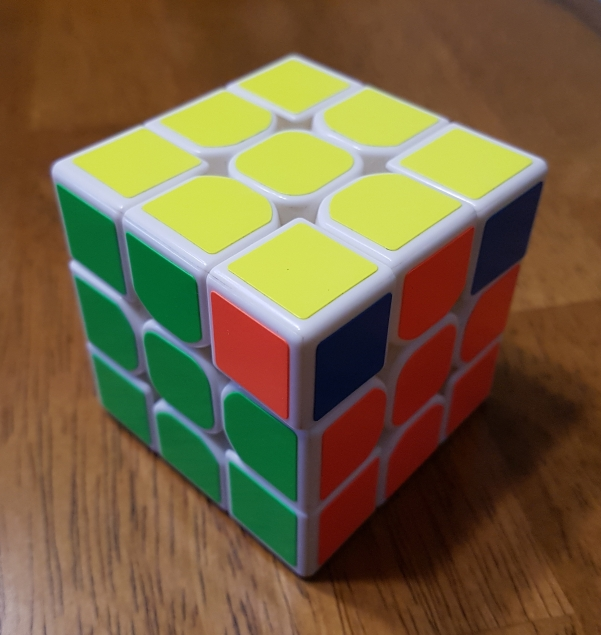(Note: the third corner piece affected is the ULB piece, or the one with the yellow sticker that is opposite to the one closest to the camera.)

It turns out that this algorithm can be generalized to any dimension $$n \ge 3$$. In turn, we can use variants of this algorithm to perform N-dimensional “monoflips” and “corner twists”. When combined with the N-dimensional A-perm, these algorithms altogether allow for solving a $$3^n$$ puzzle of arbitrary dimension $$n$$.

### A Seven-Dimensional Example

In particular, we demonstrate how the algorithm can be generalized to perform permutations of specific pieces on the seven-dimensional Rubik’s cube, or $$3^7$$ for short. The software used in this article is written by the late Andrey Astrelin, and can be found here.

Firstly, we translate the regular A-perm onto the $$3^7$$ Rubik’s cube by turning it as a $$3^4$$ Rubik’s cube, with B, F, and R replaced with BU, FU, and RU, respectively:(Note: for help on the “BU, FU, RU” notation, check the page on RKT)

Many of the pieces moved, and it seems the algorithm has moved too many pieces to be effective. However, there are different types of pieces on the puzzle, each with a different amount of stickers. We say a piece is an $$n$$-c piece (e.g. a 3c piece) if it contains $$n$$ stickers on it.

It turns out that with the regular A-perm, only three 3c pieces have moved. We see this by isolating the 3c-and-below (meaning 3c, 2c, and 1c) pieces: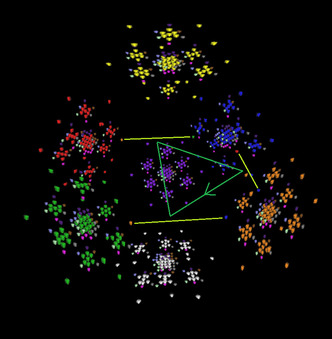Thus, we call this A-perm the A3-CW, or clockwise 3c A-perm. In general, when it comes to solving N-dimensional Rubik’s cubes, a strategy is to solve all the 2c pieces first, followed by all the 3c pieces, and so on. For reference, the 2c pieces can be solved intuitively with commutators such as the sledgehammer, sexy move, and sune, at most. We can then use these A-perms to solve the 3c-and-up pieces.

Next, if we do the moves “y A3-CW UK A3-CW’ UK’ y’”, we end up with the A4-CW, or clockwise 4c A-perm, as follows: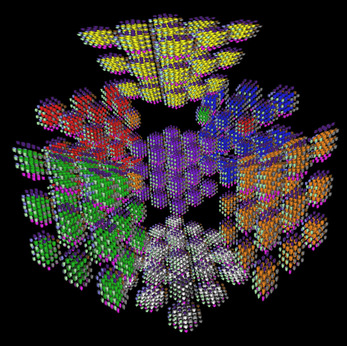Fewer pieces moved this time. In fact, none of the 3c pieces moved. If we isolate the 4c-and-below pieces, we see that only three 4c pieces moved, as desired: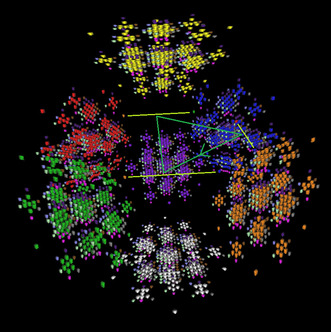For A5-CW, a UK move does not suffice, and we instead have to rotate the T cell out to a secondary face (which is possible in five dimensions and higher, using this program), do a “U turn”, and then rotate the T cell back in. The instructions are vague because we have six choices for rotating out the T cell in this case, each of which results in a variant of the A5-CW. Below is an example of one of the moves, which takes two clicks to execute:Because the second sticker clicked seems to be on the D face of that 3x3x3 block, we call the move T->D. Hence, there exist five more moves T->U, T->F, T->B, T->R, and T->L. We also note T->U’ = T->D, and so on. In fact, it is easier to execute T->D than T->U’, for the latter can only be executed in the program in 3-click mode.

We will also need a move of the form XU-shallow, where X is a side face L, R, F, or B. For reference, RU-shallow on a solved 3^7 does the following: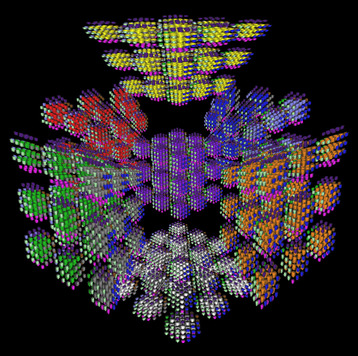It then follows that instead of UK, we do the following algorithm U*, which we customize depending on which 3x1 face of pieces we want to influence with the A5-CW:

• U* = T->U UK T->D results in an A5-CW on the D blocks
• U* = T->D UK T->U results in an A5-CW on the U blocks
• U* = T->R RU-shallow T->L results in an A5-CW on the F blocks
• U* = T->L RU-shallow T->R results in an A5-CW on the B blocks
• U* = T->F FU-shallow T->B results in an A5-CW on the L blocks
• U* = T->B FU-shallow T->F results in an A5-CW on the R blocks

Then, “y A4-CW U* A4-CW’ U*’ y’” gives us the A5-CW on a block that depends on the choice of U*. For example, if we choose U* = T->F FU-shallow T->B, then by the list above, we obtain an A5-CW on the L 3x1 blocks, as follows: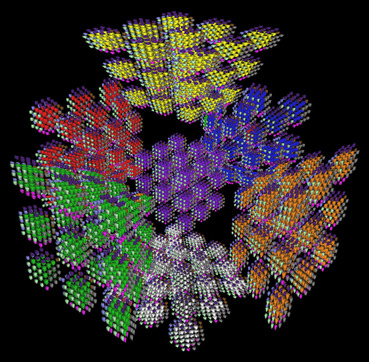Note that technically, we do some 3-cycle on the B blocks, but due to the y’ rotation at the end, we consider this A5-CW to be one that moves around the L blocks, instead.

In a similar fashion as the A5-CW, the A6-CW (on the LF edge) and A7-CW (on the URF corner) are attained, respectively: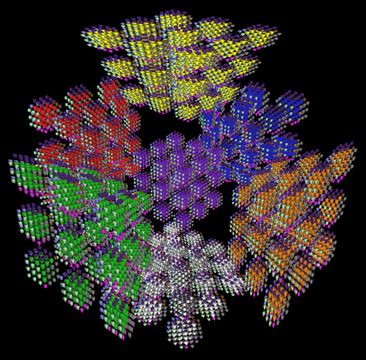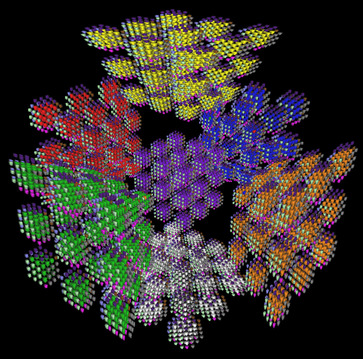And of course, here again are the A5-CW, A6-CW, and A7-CW, respectively, with arrows to indicate which 5c, 6c, and 7c pieces actually moved: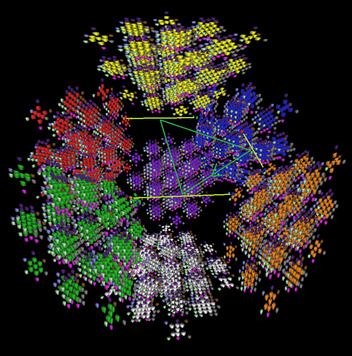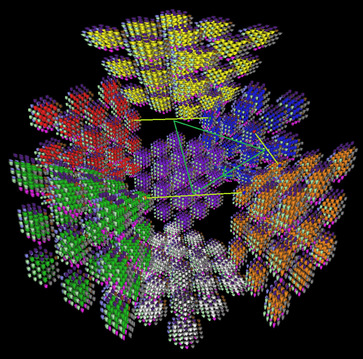Due to all the options of U*, there are 6 possible A5-CWs, 12 possible A6-CWs, and 8 possible A7-CWs based on varying the choices for U* alone. I have only shown one of each. Knowing which U* to use on each step to obtain a desired $$A_n$$-CW is important, for the N-dimensional monoflip and N-dimensional corner twists rely on executing $$A_n$$-CW’s on different blocks. However, considering how the algorithms all use y rotations, and are recursive, this task is non-trivial.

### Movecount Analysis

The regular A-perm takes 12 moves using a quarter-turn metric (QTM). The A4-CW then takes 12(2) + 1(2) = 26 turns, and the A5-CW takes 26(2) + 3(2) = 58 turns. It follows that the $$A_n$$-CW takes $$2A_{n-1} + 6$$ turns, for $$5 \le n \le 7$$. For $$n \ge 8$$, the recurrence holds if the setup moves between the $$A_{n-1}$$-CW/CCWs are still only three moves long, and more work is needed here to firmly establish whether that is the case. Nevertheless, we still have that the number of moves required for the $$A_n$$-CW is at least double of that required for the $$A_{n-1}$$-CW, and so the movecount of the $$A_n$$-CW is of order $$\Omega(2^n)$$.

In other words, the move count of the $$A_n$$-CW grows (at best) exponentially with respect to $$n$$. In particular, the A6-CW takes 122 moves, and the A7-CW takes exactly 250 moves!

Recall a piece is an $$n$$-c piece (e.g. a 3c piece) if it contains $$n$$ stickers on it. Considering that the number of $$n$$-c pieces in an $$n$$-dimensional Rubik’s cube is exactly $$2^n$$, the number of moves needed to solve the $$n$$-dimensional Rubik’s cube using commutators likely grows at best exponentially, too.

Therefore, though the $$n$$-dimensional A-perms do provide a solution to the $$N$$-dimensional Rubik’s cube by slowly permuting $$n$$-c pieces of increasing $$n$$ where $$3 \le n \le N$$, the solution becomes exponentially more tedious as $$N$$ increases, serving as a disincentive to those wanting to attempt these higher-dimensional puzzles.

Go back to Articles